# 01. The Introduction of Material¶

## Overview¶

Until now, the only way to render was to specify images and parameters and do a simple drawing. However, this did not allow complicated rendering such as distorting the image itself or adding specific areas.

Effekseer has materials to accomplish these complex rendering. You can use materials to specify how particles are drawn. Materials can be created in the Material Editor.

Here, let's create a material to create a complex rendering.

The effect created in this chapter

Effects created in this chapter

## Basic¶

When you open it, you can see that a simple sphere model has been drawn.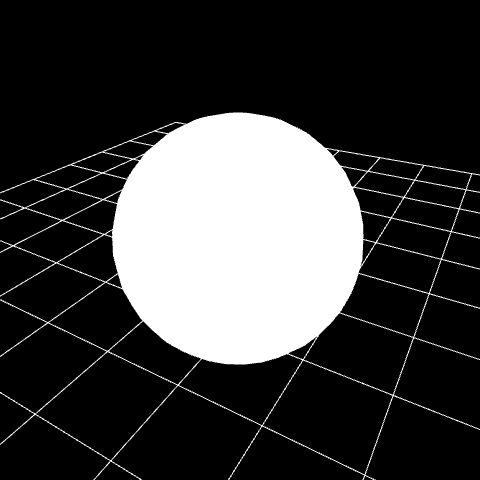In order to create a material, change `Material` to `File` in `Basic Render Settings`.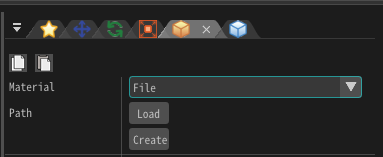Press the Create button. A dialog for creating a new material appears. When saving material is completed, the material editor starts.

Initially, only `Output` node exists. The value connected to this `Output` node is drawn on the particle.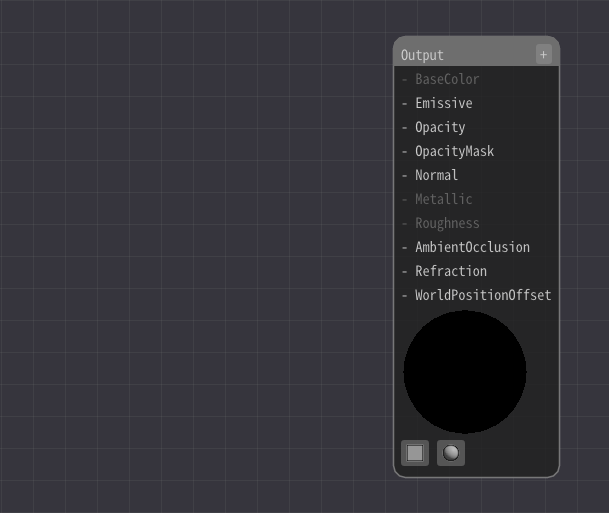In the Material Editor, connect these nodes and specify the formula for drawing. The result of the calculation is drawn as particles. It may seem difficult at first, but let's edit it little by little.

The simplest configuration is to connect `Constant3` node to the `Output` node.

Press right click to add a `Constant3` node.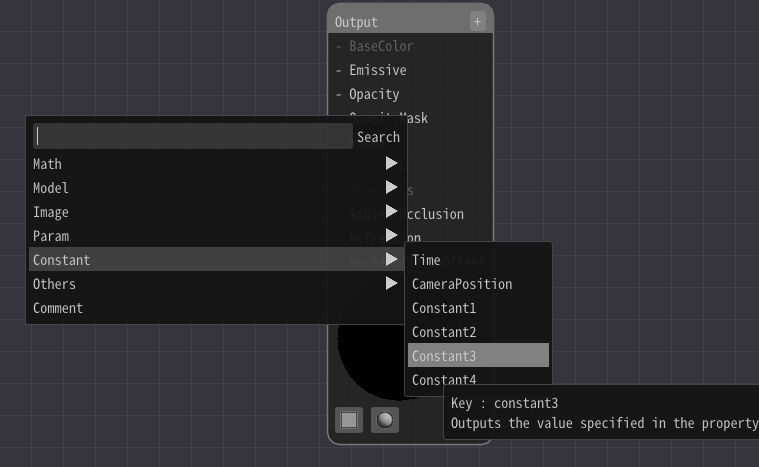Connect the `Constant3` node and `Emissive` in the `Output` node.Then, left-click on the `Constant3` node and enter a value from the input field on the left of the screen.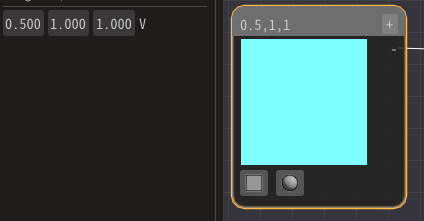Then, you can see that the color of the sphere changes according to the numerical value.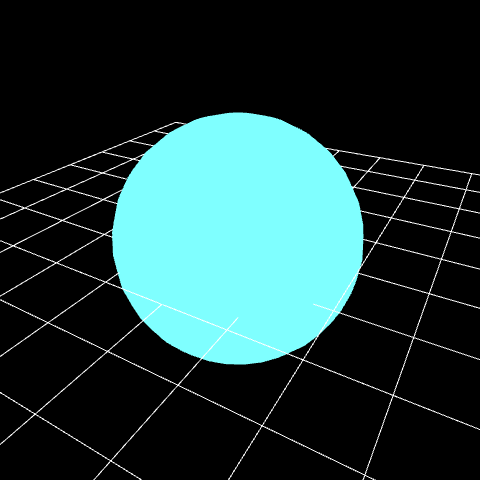When adding `Constant3` node, did you see that `Constant1-4` node existed? There are several types of values ​​that are transfered between nodes.

It is often a value type consisting of 1 to 4 numerical values, from Number 1 to Number 4. It can also be an image.

## Draw an image¶

Images can be drawn using a `Sample Image` node.

Right-click to add a `Sample Image` node.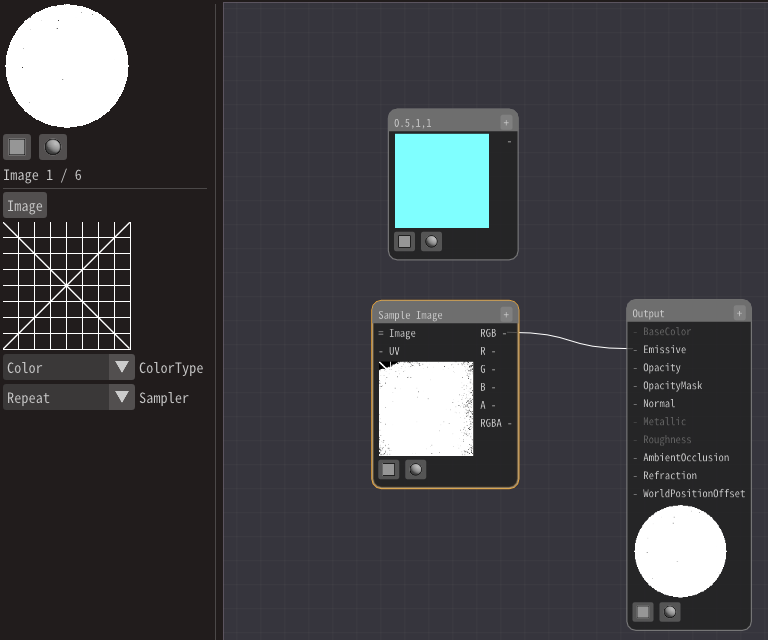Specify the image from the input field on the left of the screen. This time, specify `Textures/Grid01.png` prepared.

Connect RGB in the `Sample Image` node instead of the `Constant3` node.The image is drawn, but the transparent parts are not transparent.

Therefore, connect `A` in the `Sample Image` to `Opacity` in the `Output` node. This connects the transparency of the image to the transparency of the material.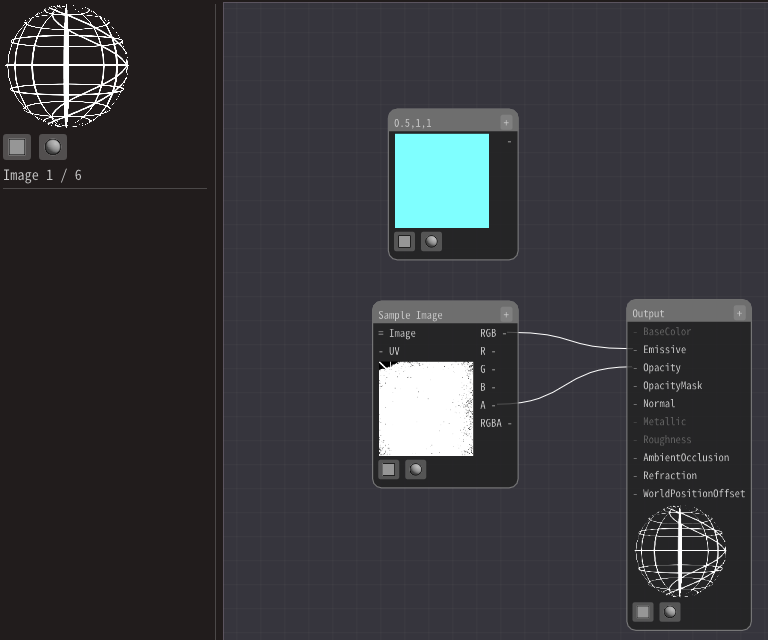Then, the image was drawn.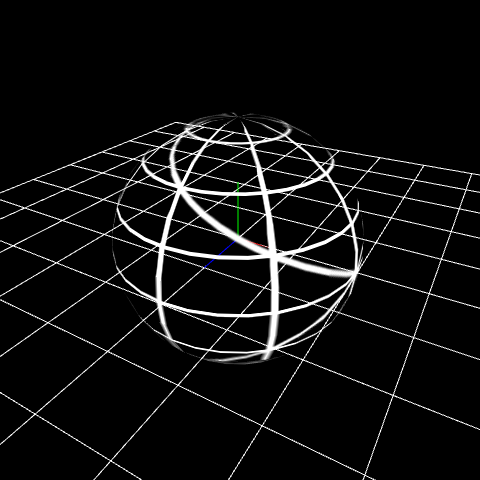## Shift an area of drawing image¶

Until now, the behavior was the same as simply drawing an image on the model. However, various expressions can be made using materials. As a preparation, change the position of the UV coordinates.

UV in the `Sample Image` is a value indicating the pixel position of the image used for draw. Let's replace this UV.

Add a `UV` node.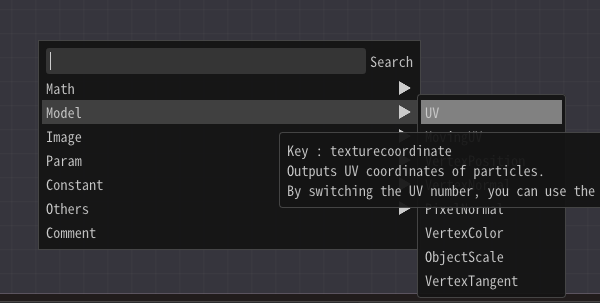Then, connect to the UV　in the `Sample Image` node.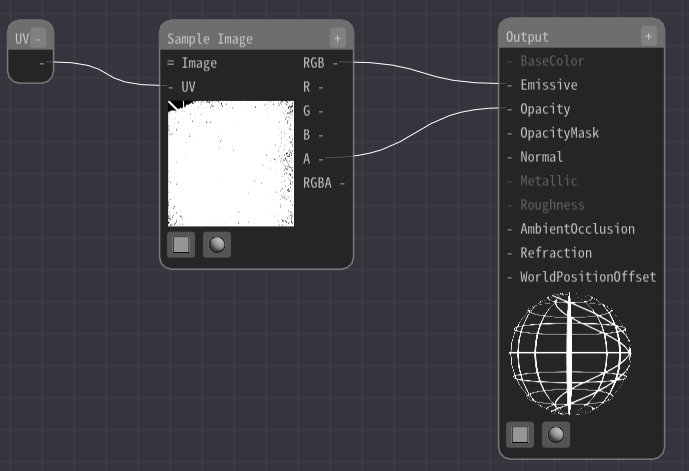Nothing changes. The reason is that a `UV` node outputs the UV value of the model or particle as it is.

Let's change this UV value and move the display area of ​​the image.

Add an `Add` node and a `Constant2` node.

Then, connect the `Constant2` node and the `UV` node to the `Add` node. Both can be connected because the value type is Number 2.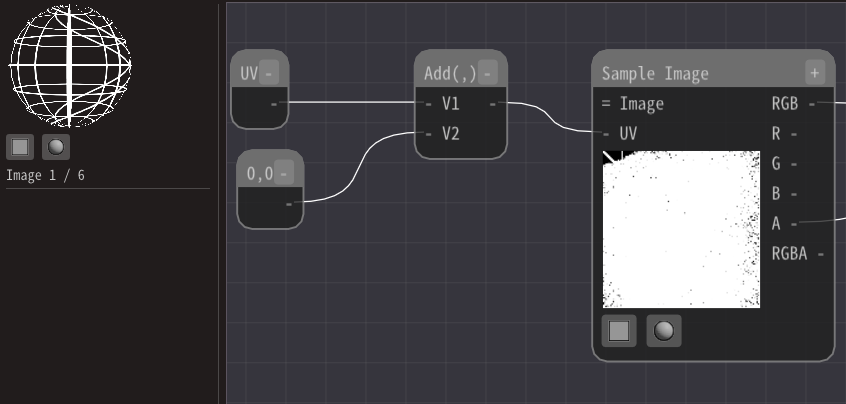Then, change the value of the `Constant2` node. Then you can see that the display position is shifted.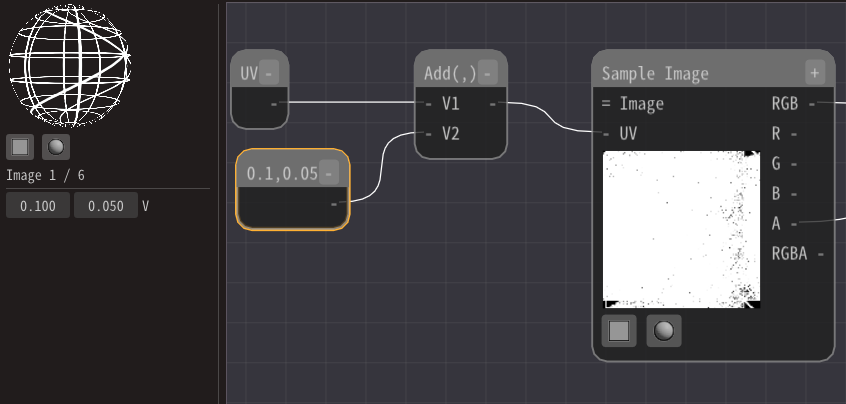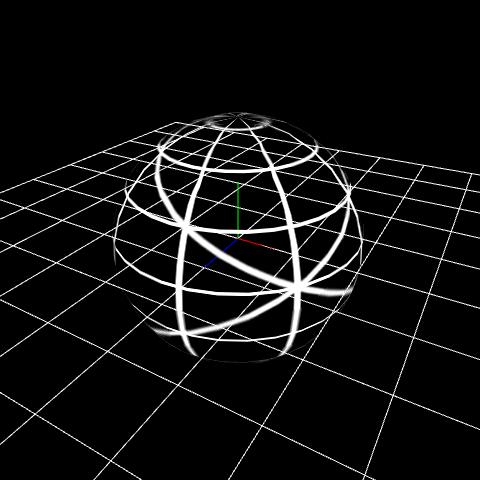## Distort the image¶

We just shifted the display area of the image, but now let's distort the image.

Use an image instead of the `Constant2` node. For the image, specify `Textures/Distortion01.png` prepared in advance.

Add a `Sample Image` node and specify the image.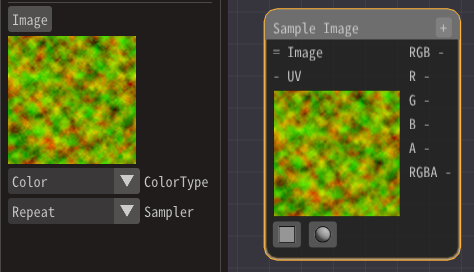Since the value type of the image is Number 3, use a `MaskElements` node.

A `MaskElements` node extracts a part of an element. Here, we will use the first two values, so check R and G.Then, connect the `MaskElements` node to the `Add` node.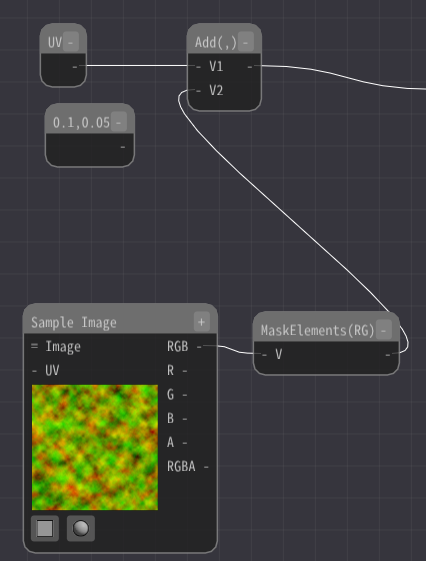Heavy distortion. But I don't want that distortion.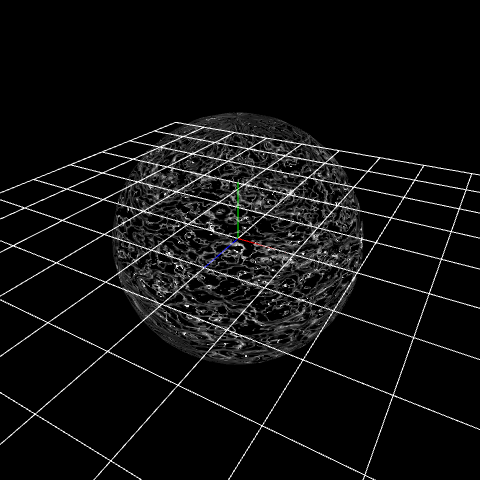Use a `Multiply` node to make it smaller. Add a `Multiply` node.

Then, connect the `MaskElements` node to the `Multiply` node. Decrease the value of the `Multiply` node.Then it will be distorted well.

## Move the distortion¶

Let's move the distortion because it is quiet when stopped.

Add a `MovingUV` node.

A `MovingUV` node outputs the value of the moving UV over time. Connect this to the `Sample Image` node. Change some parameters as well.You can see that the distortion moves.

## Color¶

Finally, let's color.

Add a `Multiply` node.

Connect the `Sample Image` node that outputs the image and the first fixed 3 nodes added to the `Multiply` node.

And connect it to Emissive.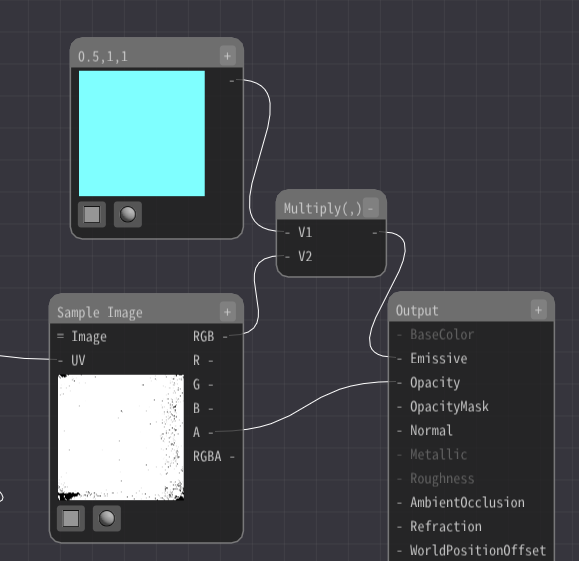Because the colors are multiplied together, they are colored.